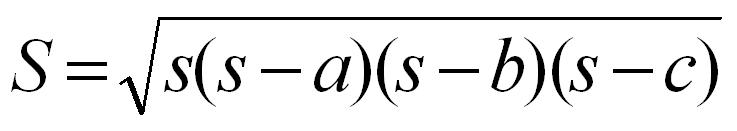2021-09-12 21:32

# 请教Java行家，初学者不是很懂• 收藏

#### 2条回答默认 最新

•已采纳

你题目的解答代码如下：（如有帮助，望采纳！谢谢! 点击我这个回答右上方的【采纳】按钮）

``````import java.util.Scanner;
import java.lang.Math;

class TriangleArea{
public static void main(String[] args) {
Scanner sc = new Scanner(System.in);
double a,b,c,s,n;
a=sc.nextDouble();
b=sc.nextDouble();
c=sc.nextDouble();
if(a+b>c && a-b<c){
s = (a+b+c)/2;
n = Math.sqrt( s*(s-a)*(s-b)*(s-c) );
System.out.println(String.format("%.3f", n));
}else{
System.out.println("不满足三角形构成条件");
}
}
}
``````
已采纳该答案
打赏 评论
•``````import java.util.Scanner;
import java.lang.Math;

public class Triangle{
public static void main(String[] args) {
Scanner input= new Scanner(System.in);

System.out.println("请输入a,b,c3条边的长度 :");
double a,b,c;
a=input.nextDouble();
b=input.nextDouble();
c=input.nextDouble();
if(a+b>c && a+c<b && a+c>b){
double p=(a+b+c)/2.0;
double s=Math.sqrt(p*(p-a)*(p-b)*(p-c));
System.out.println("面积是:"+s);
}else{
System.out.println("不能形成三角形。");
}

}
}

``````
打赏 评论